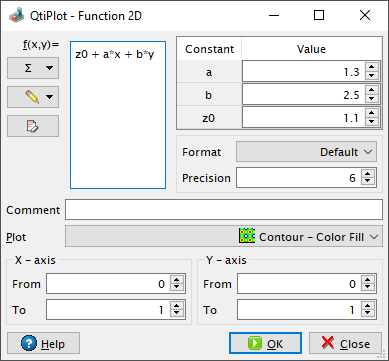# Function 2D Dialog

This dialog box is used to create a new contour/image plot window (New Function 2D... command) or to add a function of two variables (x and y) to the active plot layer (Add Function 2D... command). It is also used for editing an already existing 2D function. In the latter case it is embedded into the Plot details dialog.

Figure 5-17. The Function 2D dialog box.Using this dialog it is possible to create 2D plots from analytical functions of two variables without having to create a matrix window first. If launched as a stand-alone dialog the user may choose the type of plot to be created between the following options: Contour - Color Fill, Contour Lines - Color Mapped, Contour Lines, Gray Scale Map and Heat Map

The mathematical expression can be built with the common operators *, +, /, -, and ^ (for multiplication, addition, division, subtraction and exponentiation, respectively). A complete set of intrinsic functions are available and these are listed in the muParser section of the chapter on Mathematical Expressions and Scripting.

If a text is typed in the Comment input box, it will be used as the title of the 2D image/contour plot item.